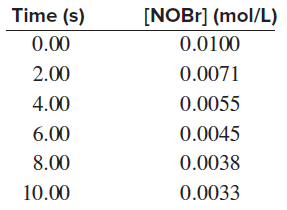# Problem: The decomposition of NOBr is studied manometrically because the number of moles of gas changes; it cannot be studied colorimetrically because both NOBr and Br2 are reddish brown:2NOBr(g) ⟶2NO(g) + Br2(g)Use the data below to answer the following:(a) Determine the average rate over the entire experiment.

🤓 Based on our data, we think this question is relevant for Professor Driessen's class at UMN.

###### FREE Expert Solution

Recall that for a reaction aA  bB, the rate of a reaction is given by:

$\overline{){\mathbf{Rate}}{\mathbf{=}}{\mathbf{-}}\frac{\mathbf{1}}{\mathbf{a}}\frac{\mathbf{\Delta }\mathbf{\left[}\mathbf{A}\mathbf{\right]}}{\mathbf{\Delta t}}{\mathbf{=}}\frac{\mathbf{1}}{\mathbf{b}}\frac{\mathbf{\Delta }\mathbf{\left[}\mathbf{B}\mathbf{\right]}}{\mathbf{\Delta t}}}$

where:

Δ[A] = change in concentration of reactants or products (in mol/L or M), [A]final – [A]initial

Δt = change in time, tfinal – tinitial###### Problem Details

The decomposition of NOBr is studied manometrically because the number of moles of gas changes; it cannot be studied colorimetrically because both NOBr and Br2 are reddish brown:

2NOBr(g) ⟶2NO(g) + Br2(g)

Use the data below to answer the following:

(a) Determine the average rate over the entire experiment.